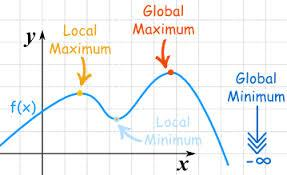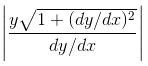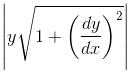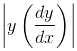Courses

# Notes | EduRev

## JEE : Notes | EduRev

The document Notes | EduRev is a part of the JEE Course JEE Main Mock Test Series 2020 & Previous Year Papers.
All you need of JEE at this link: JEE
• The concepts of straight line, maxima and minima, global maxima and minima, Rolle’s Theorem and LMVT all come under the head of Application of Derivatives.
• If a function is increasing on some interval then the slope of the tangent is positive at every point of that interval due to which its derivative is positive.
• Similarly, the derivative of a function which is decreasing on some interval is negative as the slope of the tangent is negative at every point of that interval.• A function f is said to have a local maximum (also termed as relative maximum) at x = a if f(x) ≤ f(c), for every x in some open interval around x = c.
• A function f is said to have a relative minimum or a local minimum around x = c if f(x) ≥ f(c), for every x in some open interval around x = a.
• A function f is said to have a global maximum (also termed as absolute maximum) at x = a if f(x) ≤ f(c), for every x in the domain under consideration.

A function f is said to have an absolute minimum or a global minimum around x = c if f(x) ≥ f(c), for every x in the whole domain under consideration.

 Rolle’s Theorem

Let y = f (x) be a given function which satisfies the conditions:
1) f (x) is continuous in [a , b]
2) f (x) is differentiable in (a , b)
3) f (a) = f (b)

Then f'(x) = 0 at least once for some x ∈ (a, b).

• Certain points to be noted in Rolle’sTheorem include:
• Converse of the theorem does not hold good.
• There can be more than one such c.
• The conditions of Rolle’s Theorem are only sufficient and not necessary.
 Lagrange Mean Value Theorem (LMVT)
• If a given function y = f (x) satisfies certain conditions like:
f(x) is continuous in [a , b]
f(x) is differential in (a, b)
then f'(x) = [f(b) – f(a)]/[b–a] for some x ∈ (a, b). This is the generalization of the Rolle’s Theorem and is termed as Lagrange Mean Value theorem.
• A function is said to be monotonically increasing at x = a if f(x) satisfies f(a+h) > f(a) and f(a-h) < f(a), for some small positive h.
• A function is said to be monotonically decreasing at x = a if f(x) satisfies f(a+h) < f(a) and f(a-h) > f(a), for some small positive h.
• If f'(x) > 0 ∀ x ∈ (a,b) and points which make equal to zero (in between (a, b)) don’t form an interval, then f (x) would be increasing in [a, b] otherwise it will be non-decreasing function.
• If f'(x) > 0 ∀ x ∈ (a,b) and points which make equal to zero (in between (a, b)) don’t form an interval, f (x) would be decreasing in [a, b], otherwise it will be non-increasing.
• For all x and y, such that x≤ y, if f(x) ≤ f(y), then the function f is said to be monotonically increasing, increasing or non-decreasing.
• Similarly, for x ≤ y, if f(x) ≥ f(y), then the function is monotonically decreasing, decreasing or non-increasing i.e. it reverses the order.
• If f is increasing for x > a and f is also increasing for x < a then f is also increasing at x = a provided f(x) is continuous at x = a.
• If f(x) is strictly increasing, then f-1 exists and is also strictly increasing.
• If f(x) is strictly increasing on [a, b] and is also continuous then f-1 is continuous on [f(a), f(b)].
• If f(x) and g(x) are strictly increasing (decreasing) functions on [a, b], then gof(x) is strictly increasing (decreasing) function on [a, b].
• If one of the two functions f(x) and g(x) is strictly increasing and other is strictly decreasing then gof(x) is strictly decreasing on [a, b].
• If a continuous function y = f(x) is strictly increasing in the closed interval [a, b], then f(a) is the least value.
• If f(x) is decreasing in [a, b], then f(b) is the least and f(a) is the greatest value of f(x) in [a, b].
• If f(x) is non-monotonic in [a, b] and is continuous then the greatest and the least value of f(x) in [a, b] are those where f(x) = 0 or f’(x) does not exist or at the extreme values.
• The direction of acceleration is in the direction of velocity or opposite to it.
• When the particle is going upward, the value of g is negative and when it is coming back, the value of g is positive.
• At maximum height the velocity of a particle is zero. The value of g is 9.8 m/sor 980 cm/s2.
• Slope of tangent to the curve y = f(x) at the point (x, y) is m = tan θ = [dy/dx](x,y)
• If the equation of the curve is in the parametric form x = f(t) and y = g(t), then the equations of the tangent and the normal are y – g(t) = g'(t)/f'(t)(x–f(t)) and f'(t)[x–f(t)] + g'(t) [y–g(t)] = 0 respectively.
• The equation of tangent to the curve y = f(x) at the point P(x1, y1) is given by y – y= [dy/dx](x,y) (x – x1)
• If dy/dx = 0 then the tangent to curve y = f(x) at the point (x, y) is parallel to the x-axis.
• If dy/dx → ∞, dx/dy = 0, then the tangent to the curve y = f(x) at the point (x, y) is parallel to the y-axis.
• If dy/dx = tan θ > 0, then the tangent to the curve y = f(x) at the point (x, y) makes an acute angle with positive x-axis and vice versa.
• If two curves are orthogonal, then the product of their slopes is -1 everywhere wherever they intersect.
• Length of tangent, normal, subtangent, subnormal:
Tangent =Subtangent =Normal =Subnormal =Offer running on EduRev: Apply code STAYHOME200 to get INR 200 off on our premium plan EduRev Infinity!

## JEE Main Mock Test Series 2020 & Previous Year Papers

3 videos|174 docs|151 tests

,

,

,

,

,

,

,

,

,

,

,

,

,

,

,

,

,

,

,

,

,

;